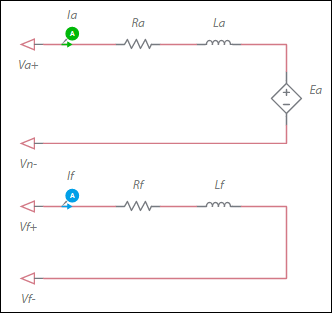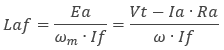OK

# DC machine wound field model

Model assumptions

• Linear iron magnetization with no saturation in both the field and armature winding
• Uniform air-gap
• Uniform torque profile

Configuration of the machine electrical systemEa = ωm * If * Laf

where

Ea = back-EMF voltage
ωm = Mechanical angular speed
Laf = Armature-field mutual inductance
If = Field current

The generated electromagnetic torque is:

Te = Ia * If * Laf

Parameter Description
Armature inductance Inductance of the armature winding.
Armature resistance Ra in the equation in Deriving Laf from datasheets, below.
Field winding inductance Field inductance.
Field winding resistance. Field resistance.
Armature-field mutual inductance See Deriving Laf from datasheets, below.
Shaft inertia Inertia of the shaft in kg•m<sup>2</sup>. J<sub>rotor</sub> on the machine model diagram in <a href="/help/components/machine-modeling/">Machine modeling</a>.
Shaft friction This is F<sub>rotor</sub> on the machine model diagram in <a href="/help/components/machine-modeling/">Machine modeling</a>.
Initial angular speed Rotational measurement of the shaft angle in rad/s at the start of the simulation.
Initial angle Initial shaft angle in radians.

Deriving Laf from datasheets

Rearranging the back-EMF equation, Laf can be expressed as:where

Laf = Armature-field mutual inductance
Ea = Back-EMF voltage
Vt = Terminal voltage applied to the machine